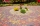# A bridge

A bridge over a river is in the shape of the arc of a circle with each base of the bridge at the river's edge. At the center of the river, the bridge is 10 feet above the water. At 27 feet from the edge of the river, the bridge is 9 feet above the water. How wide is the river?

Result

a =  80 ft

#### Solution:Checkout calculation with our calculator of quadratic equations.

Leave us a comment of example and its solution (i.e. if it is still somewhat unclear...):

Showing 2 comments:Dr Math
THE BRIDGE OVER THE RIVERDr Math
r = radius of circle of arc

x = distance between center of circle and water level (center of the circle is under ground)

y = distance in horiznotal of point on circle which is 9 ft above the water#### To solve this example are needed these knowledge from mathematics:

Looking for help with calculating roots of a quadratic equation? Do you have a system of equations and looking for calculator system of linear equations? Pythagorean theorem is the base for the right triangle calculator. See also our trigonometric triangle calculator.

## Next similar examples:

1. PavementCalculate the length of the pavement that runs through a circular square with a diameter of 40 m if distance the pavement from the center is 15 m.
2. RT and circlesSolve right triangle if the radius of inscribed circle is r=9 and radius of circumscribed circle is R=23.
3. Circle annulusThere are 2 concentric circles in the figure. Chord of larger circle 10 cm long is tangent to the smaller circle. What are does annulus have?
4. Three unknownsSolve the system of linear equations with three unknowns: A + B + C = 14 B - A - C = 4 2A - B + C = 0
5. Linsys2Solve two equations with two unknowns: 400x+120y=147.2 350x+200y=144
6. Expression with powersIf x-1/x=5, find the value of x4+1/x4
7. Three workshopsThere are 2743 people working in three workshops. In the second workshop works 140 people more than in the first and in third works 4.2 times more than the second one. How many people work in each workshop?
8. Isosceles triangleThe leg of the isosceles triangle is 5 dm, its height is 20 cm longer than the base. Calculate base length z.
9. DiscriminantDetermine the discriminant of the equation: ?
10. EquationEquation ? has one root x1 = 8. Determine the coefficient b and the second root x2.
11. RootsDetermine the quadratic equation absolute coefficient q, that the equation has a real double root and the root x calculate: ?
12. AlgebraX+y=5, find xy (find the product of x and y if x+y = 5)
13. Elimination methodSolve system of linear equations by elimination method: 5/2x + 3/5y= 4/15 1/2x + 2/5y= 2/15
14. Quadratic equationFind the roots of the quadratic equation: 3x2-4x + (-4) = 0.
15. Theorem proveWe want to prove the sentense: If the natural number n is divisible by six, then n is divisible by three. From what assumption we started?
16. LegsCancer has 5 pairs of legs. The insect has 6 legs. 60 animals have a total of 500 legs. How much more are cancers than insects?
17. Holidays - on poolChildren's tickets to the swimming pool stands x € for an adult is € 2 more expensive. There was m children in the swimming pool and adults three times less. How many euros make treasurer for pool entry?